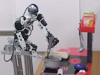### Abstract

In manipulation tasks, motion trajectories are characterized by a set of key phases (i.e., motion primitives). It is therefore important to learn the motion primitives embedded in such tasks from a complete demonstration. In this paper, we propose a core framework that autonomously segments motion trajectories to support the learning of motion primitives. For this purpose, a set of segmentation points is estimated using a Gaussian Mixture Model (GMM) learned after investigating the dimensional subspaces reduced by Principal Component Analysis. The segmentation points can be acquired by two alternative approaches: (1) using a geometrical interpretation of the Gaussians obtained from the learned GMM, and (2) using the weights estimated along the time component of the learned GMM. The main contribution of this paper is the autonomous estimation of the segmentation points based on the GMM learned in a reduced dimensional space. The advantages of such an estimation are as follows: (1) segmentation points without any internal parameters to be manually predefined or pretuned (according to the types of given tasks and/or motion trajectories) can be estimated from a single training data, (2) segmentation points, in which non-linear motion trajectories can be better characterized than by using the original motion trajectories, can be estimated, and (3) natural motion trajectories can be retrieved by temporally rearranging motion segments. The capability of this autonomous segmentation framework is validated by four experiments. In the first experiment, motion segments are evaluated through a comparison with a human expert using a publicly available kitchen dataset. In the second experiment, motion segments are evaluated through a comparison with an existing approach using an open handwriting database. In the third experiment, the segmentation performance is evaluated by retrieving motion trajectories from the reorganization of motion segments. In the fourth experiment, the segmentation performance is evaluated by clustering motion segments.

### Bibtex reference

@article{Lee15,
author="Lee, S. H. and Suh, I. H. and Calinon, S. and Johansson, R.",
title="Autonomous Framework for Segmenting Robot Trajectories of Manipulation Task",
journal="Autonomous Robots",
publisher="Springer",
year="2015",
month="February",
volume="38",
number="2",
pages="107--141"
}